# Section 5 Lists again Double linked lists insertion

• Slides: 19Section 5 • Lists again. • Double linked lists – insertion, deletion. • Trees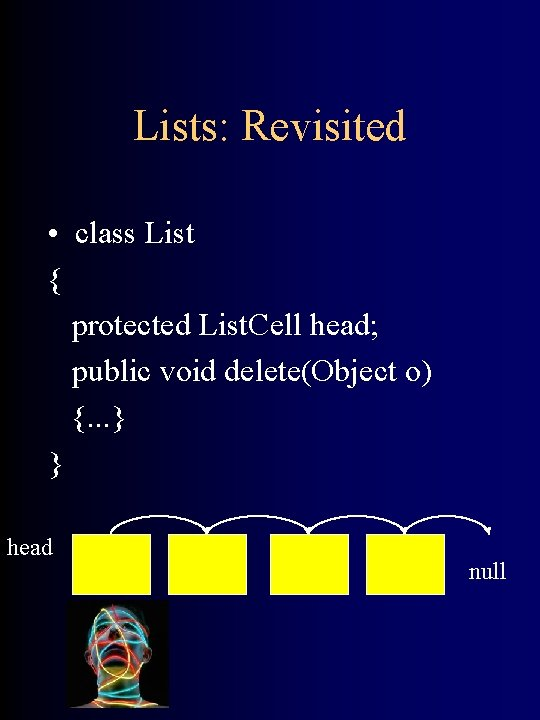Lists: Revisited • class List { protected List. Cell head; public void delete(Object o) {. . . } } head nullList cells: • Cells containg Objects. Each Cell has a pointer to the next cell in the list. class List. Cell {. . . public List. Cell get. Next(); // returns the next element. public void set. Next(List. Cell l); // sets the pointer to point to l public Object get. Datum(); // returns the object stored in the cell }Deleting • Iterative version: public void delete(Object o) { List. Cell current = head, previous = null; while (current != null) { if (current. get. Datum(). equals(o)) // found the object { if (previous == null) return l. get. Next() ; // it was the first one else { previous. set. Next(current. get. Next()); return l; } } else previous = current; current = current. get. Next(); } }Deleting 2: Revisited • Deleting element: recursive way: Intuition: • – If list l is empty, return null. • – If first element of l is o, return rest of list l. • – Otherwise, return list consisting of first element of l, and the list that results from deleting o from the rest of list l.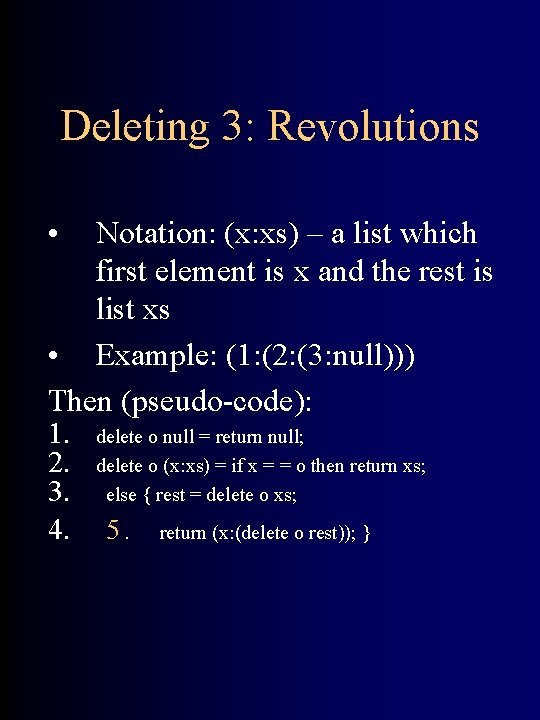Deleting 3: Revolutions • Notation: (x: xs) – a list which first element is x and the rest is list xs • Example: (1: (2: (3: null))) Then (pseudo-code): 1. delete o null = return null; 2. delete o (x: xs) = if x = = o then return xs; 3. else { rest = delete o xs; 4. 5. return (x: (delete o rest)); }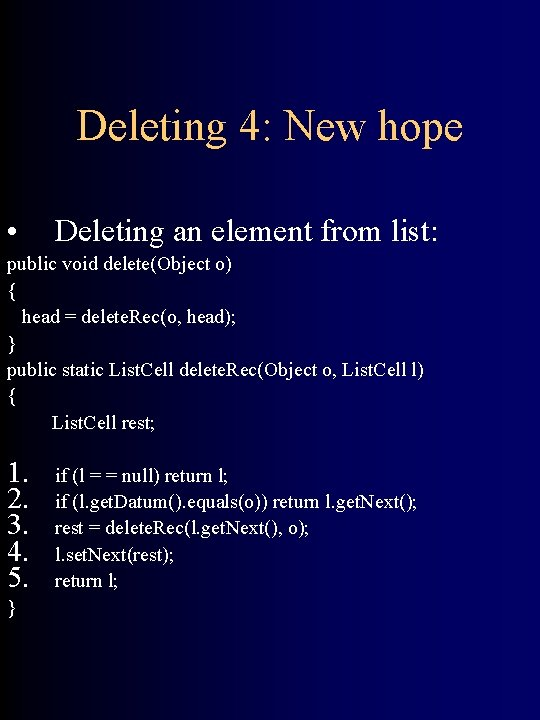Deleting 4: New hope • Deleting an element from list: public void delete(Object o) { head = delete. Rec(o, head); } public static List. Cell delete. Rec(Object o, List. Cell l) { List. Cell rest; 1. 2. 3. 4. 5. } if (l = = null) return l; if (l. get. Datum(). equals(o)) return l. get. Next(); rest = delete. Rec(l. get. Next(), o); l. set. Next(rest); return l;Doubly-linked lists class DLLCell { protected Object datum; protected DLLCell next; protected DLLCell previous; …. . }Doubly-linked lists • class Dlist { protected DLLCell head; public void insert. After(Object o, Object a) // inserts o after a { insert. After. Rec(head, o, a); } public void delete(Object o); }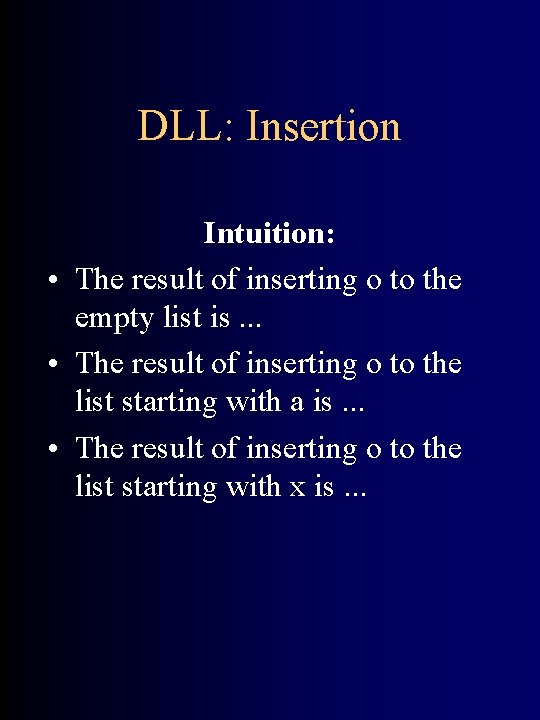DLL: Insertion Intuition: • The result of inserting o to the empty list is. . . • The result of inserting o to the list starting with a is. . . • The result of inserting o to the list starting with x is. . .DLL: Insertion Intuition: • The result of inserting o to the empty list is a list containing o. • The result of inserting o to the list starting with a is a list containing a, o and the rest of original list. • The result of inserting o to the list starting with x is the list containing x and the result of inserting o to the rest of the original list.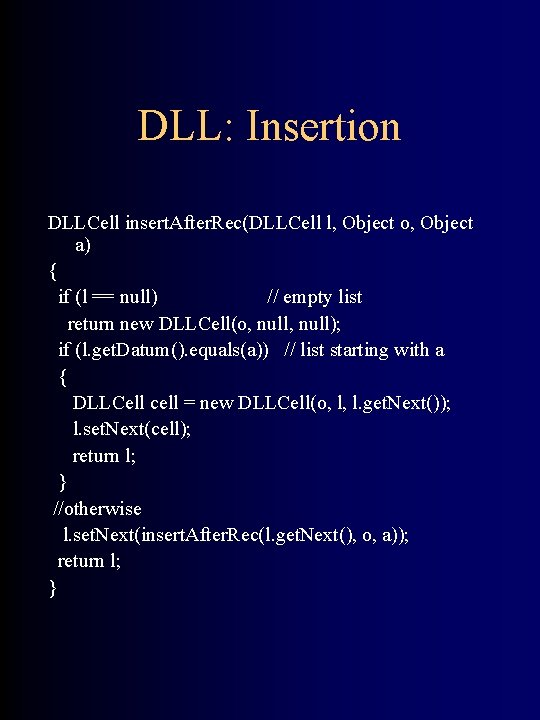DLL: Insertion DLLCell insert. After. Rec(DLLCell l, Object o, Object a) { if (l == null) // empty list return new DLLCell(o, null); if (l. get. Datum(). equals(a)) // list starting with a { DLLCell cell = new DLLCell(o, l, l. get. Next()); l. set. Next(cell); return l; } //otherwise l. set. Next(insert. After. Rec(l. get. Next(), o, a)); return l; }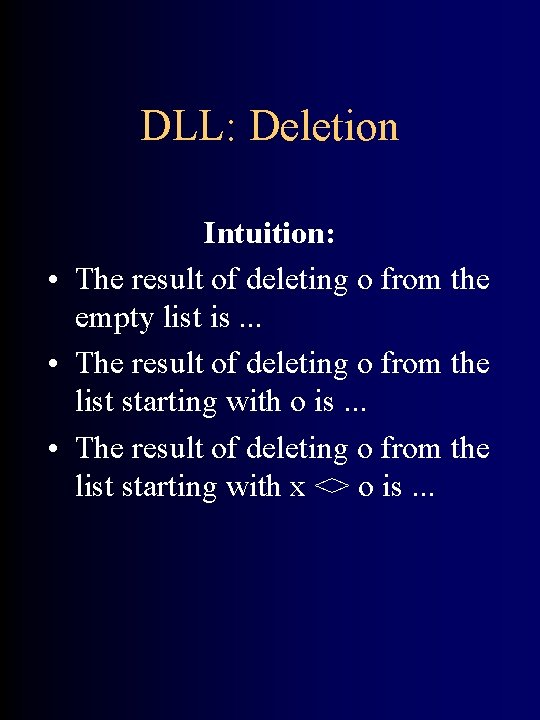DLL: Deletion Intuition: • The result of deleting o from the empty list is. . . • The result of deleting o from the list starting with o is. . . • The result of deleting o from the list starting with x <> o is. . .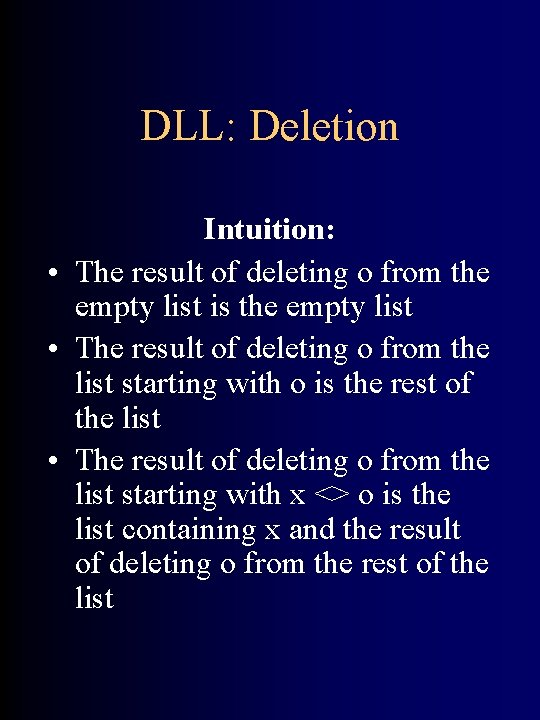DLL: Deletion Intuition: • The result of deleting o from the empty list is the empty list • The result of deleting o from the list starting with o is the rest of the list • The result of deleting o from the list starting with x <> o is the list containing x and the result of deleting o from the rest of the list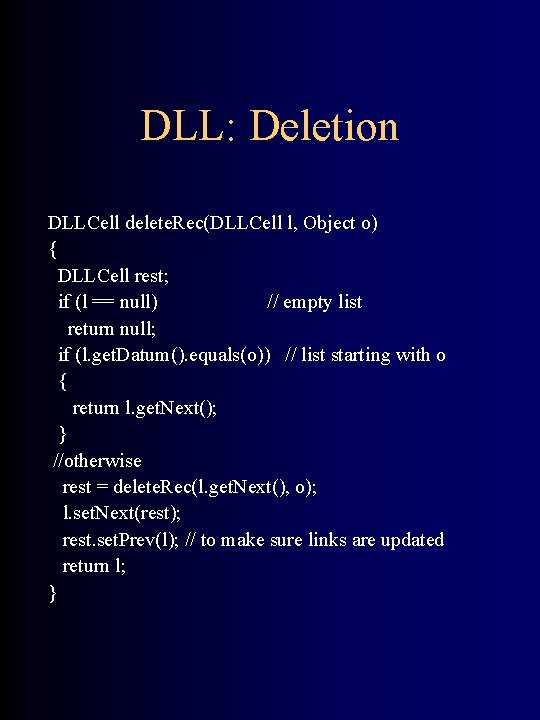DLL: Deletion DLLCell delete. Rec(DLLCell l, Object o) { DLLCell rest; if (l == null) // empty list return null; if (l. get. Datum(). equals(o)) // list starting with o { return l. get. Next(); } //otherwise rest = delete. Rec(l. get. Next(), o); l. set. Next(rest); rest. set. Prev(l); // to make sure links are updated return l; }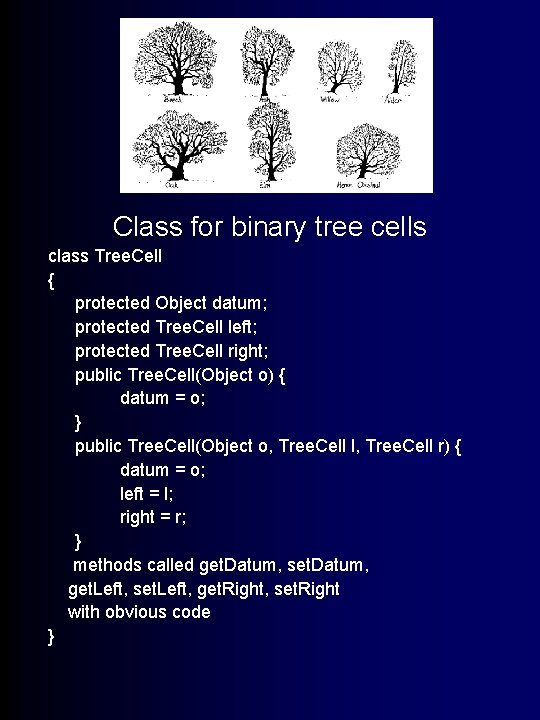Trees! Class for binary tree cells class Tree. Cell { protected Object datum; protected Tree. Cell left; protected Tree. Cell right; public Tree. Cell(Object o) { datum = o; } public Tree. Cell(Object o, Tree. Cell l, Tree. Cell r) { datum = o; left = l; right = r; } methods called get. Datum, set. Datum, get. Left, set. Left, get. Right, set. Right with obvious code }Height of a tree • Intuition: • The height of a an empty tree is -1 • The height of a tree containing leaf is. . . • The height of a tree containing subtrees l 1 and l 2 is. . .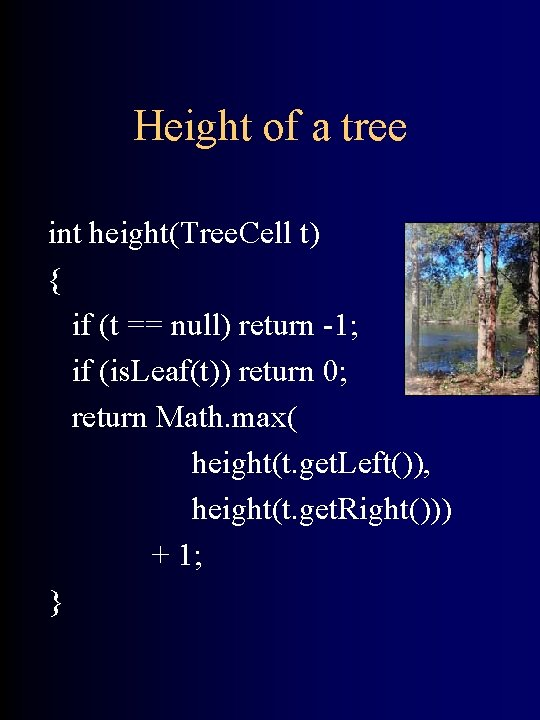Height of a tree int height(Tree. Cell t) { if (t == null) return -1; if (is. Leaf(t)) return 0; return Math. max( height(t. get. Left()), height(t. get. Right())) + 1; }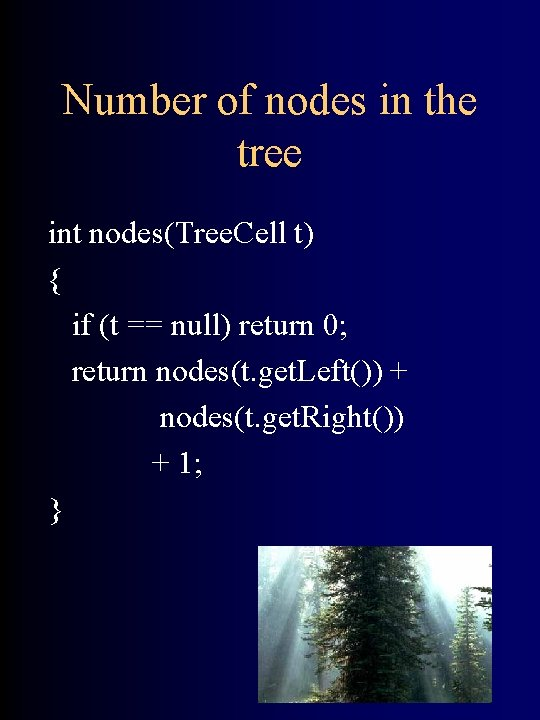Number of nodes in the tree int nodes(Tree. Cell t) { if (t == null) return 0; return nodes(t. get. Left()) + nodes(t. get. Right()) + 1; }